# 面试中常见的 C 语言与 C++ 区别的问题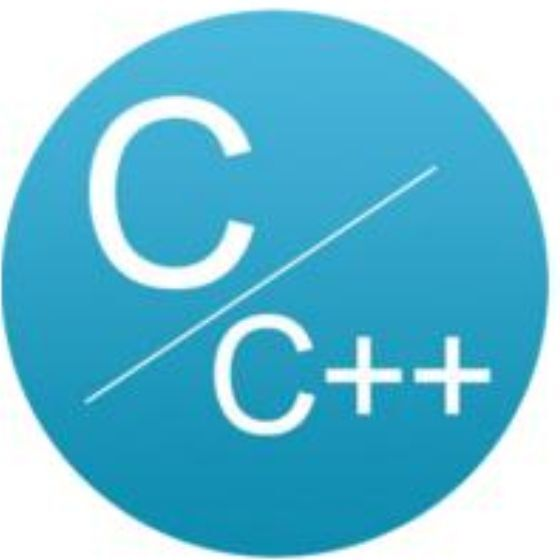C语言与CPP编程

## C 和 C++的区别

• C 语言是一种结构化语言，其偏重于数据结构和算法，属于过程性语言

• 虽然在语法上 C++完全兼容 C 语言，但是两者还是有很多不同之处。下面将详细讲解 C 和 C++不同之处的常见考题

## 关键字 static 在 C 和 C++区别

C 和 C++中都有关键字 static 关键字，那么 static 关键字在 C 和 C++中的使用有什么区别？请简述之。

## 1. 定义局部静态变量

• 局部静态变量存储在静态存储区，在程序运行期间都不会释放，只在声明时进行初始化，而且只能初始化一次，如果没有初始化，其自动初始化为 0 或空字符。具有局部变量的“记忆性”和生存周期“全局性”特点。

• 局部变量的“记忆性”是指在两次函数调用时，第二次调用开始时，变量能够保持上一次调用结束数的值。如下例：

#include <stdio.h>//20200505 公众号：C语言与CPP编程void staticShow(){	static int a=10;	printf("a=%d\n",a);	a += 10;}int  main(){	for(int i=0;i<4;i++)	{	  staticShow();		}	return 0;}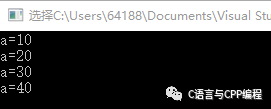#include <stdio.h>#include <string.h>//20200505 公众号：C语言与CPP编程char *p = NULL;char *helloToStr(char *b){	static char a;	a='H';	a='E';	a='L';	a='L';	a='O';	strcpy(a+5,b);	p=a;	return a;};int main(void){	printf("%s\n",helloToStr("yang"));    	strcpy(p+5,"song");	printf("%s\n",p);		strcpy(p+5,"zhang");	printf("%s\n",p);	strcpy(p+5,"wang");	printf("%s\n",p);	return 0;}## 2.限定访问区域

//Test1.cppstatic int a;int b;extern void fun1(){	......}static void fun1(){	......}//Test2.cppextern int a;        //错误，a是static类型，无法在Test2.cpp文件中使用extern int b;       //使用Test1.cpp中定义的全局变量extern void fun1();    //使用Test1.cpp中定义的函数extern void fun2();    //错误，无法使用Test1.cpp文件中static函数

#include <iostream.h>#include <string.h>class A{public:	static int a;	static int geta();	int b;	int getb();};int A::a=100;int A::geta(){	return a;}int A::getb(){	return b;}int  main(void){	A m,n;	m.b=90;	cout<<m.geta()<<endl;	cout<<m.getb()<<endl;	cout<<m.a<<endl;	n.a=33;	n.b=44;	cout<<m.geta()<<endl;	cout<<m.getb()<<endl;	cout<<m.a<<endl;	return 0;}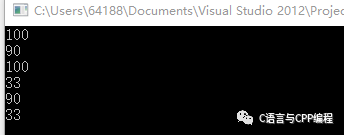## 结构体在 C 语言和 C++的区别

#include <iostream.h>struct A{public:	int a;	int gata()	{    	return a;	}};int  main(void){ m.a=50; cout<<m.gata()<<endl; return 0;}

## 答案

• C 语言的结构体是不能有函数成员的，而 C++的类可以有。

• C 语言结构体中数据成员是没有 private、public 和 protected 访问限定的。而 C++的类的成员有这些访问限定（在 C++中结构体的成员也是有访问权限设定的，但是类成员的默认访问属性是 private，而结构体的默认访问属性是 public）。

• C 语言的结构体是没有继承关系的，而 C++的类却有丰富的继承关系。

## C 中 malloc 和 C++的 new 区别

• malloc、free 是标准库函数，new、delete 则是运算符。malloc、free 在 C、C++中都可使用，而 new、delete 只属于 C++。

• malloc 要指定申请内存的大小，其申请的只是一段内存空间。而 new 不必指定申请内存的大小，建立的是一个对象。

• new、delete 在申请非内部数据类型的对象时，对象在创建的同时会自动执行构造函数，在消亡时会自动执行析构函数，这不在编译器的控制之内，所以 malloc、free 无法实现。

• new 返回的是某种数据类型的指针，而 malloc 返回的是 void 型指针。

• 由于 new、delete 是运算符，可以重载，不需要头文件的支持，而 malloc、free 是库函数，可以覆盖，并且要包含相应的头文件。

## 答案

1. new、delete 是操作符，可以重载，只能在 C++中使用。

2. malloc、free 是函数，可以覆盖，C、C++中都可以使用。

3. new 可以调用对象的构造函数，对应的 delete 调用相应的析构函数。

4. malloc 仅仅分配内存，free 仅仅回收内存，并不执行构造和析构函数。

5. new、delete 返回的是某种数据类型指针，malloc、free 返回的是 void 指针。

## 1、作为函数的参数

void swap(int *a, int *b){	int temp;	temp = *a;    *a = *b;	*b = temp;}int  main(void){	int a=10,b=5;	cout<<"Before change:"<< a<<""<<b<<endl;	swap(&a,&b);	cout<<"After change:"<< a<<""<<b<<endl;	return 0;}

Before change: 10 55

After change: 55 10

void swap(int &a, int &b){	int temp;	temp = a;        a = b;	b = temp;}int  main(void){	int a=10,b=5;	cout<<"Before change:"<< a<<""<<b<<endl;	swap(a,b);	cout<<"After change:"<< a<<""<<b<<endl;	return 0;}

## 2、引用作为函数的返回值

1. 指针可不初始化且初始化的时候，可以指向一个地址，也可以为空。引用必须初始化且只能初始化为另一个变量，如下：

int a=1024;int *p=&a;int &b=a;

int a=1,b=2;int *p1=&a, *p2=&b;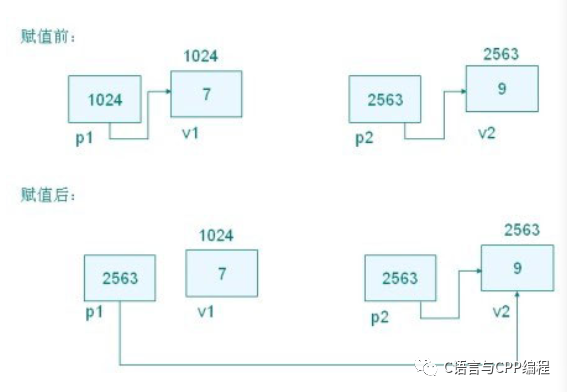int a=1,b=2;int &v1=a, &v2=b;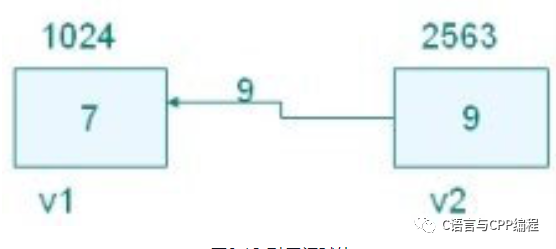1. 指针可以被重新赋值以指向另一个不同的对象。但是引用则总是指向在初始化时被指定的对象，以后不能改变。如下所示：

int a=1;int b=2;int &v1=a;      //v1引用astring *pi = &a; //pi指向av1=b;             //v1仍旧引用a，但是a现在的值是2；pi=&b;             //pi指向b，a没有改变

## 答案

• 引用必须被初始化，但是不分配存储空间。指针不声明时初始化，在初始化的时候需要分配存储空间。

• 引用初始化以后不能被改变，指针可以改变所指的对象。

• 不存在指向空值的引用，但是存在指向空值的指针。## 评论